# Different Math Course Difficulties Between Teachers

in Education9 months ago

Hi everyone. In this math education themed post, I would like to talk about an issue that came to my attention recently. It is about math teachers having different course difficulties even though they are teaching the same math course.

When I refer to teachers, I mainly refer to high school math teachers. Some of the content here can apply to teachers in generals and university professors.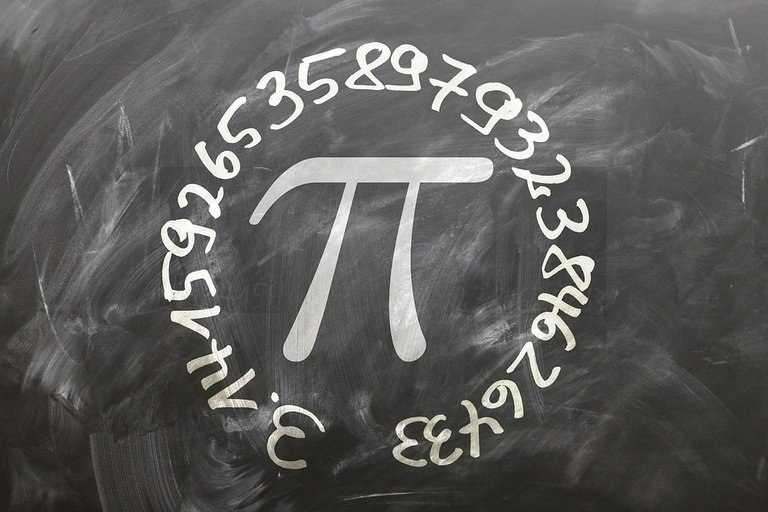Pixabay Image Source

## Math Teaching Speed Can Vary

Math teachers from a school math department typically have a course schedule to follow. There are cases where math teachers do not follow the course schedule properly. Some math teachers can be on pace with the course schedule, some math teachers teach slower than the suggested schedule and there are some math teachers who teach too much too fast.

In the case of a slow teaching math teacher, the slow speed can help students with learning more technical concepts. A big issue with the slow speed is not being able to teach every concept in the whole course/semester.

A fast math teacher teaching a math course ensures that all topics in the course are taught in the course. The main downside is that there could be too much content to understand and digest. A math test every week for example can be too much for students who may have other technical and demanding courses in a semester.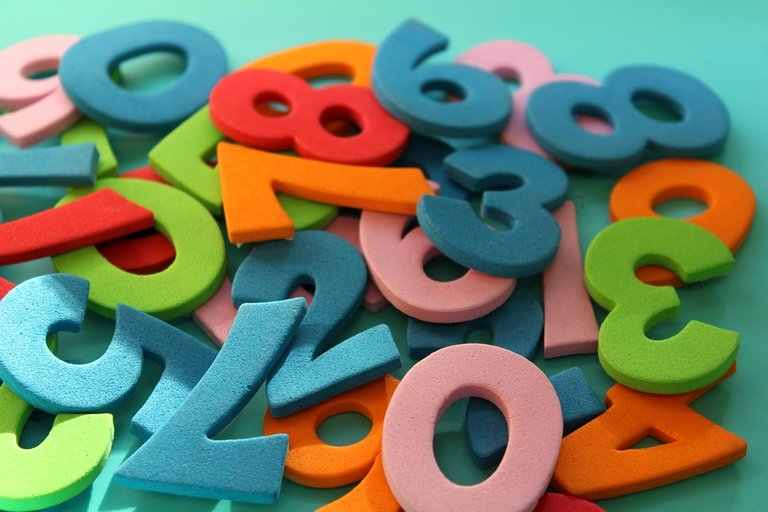Pixabay Image Source

## Tests From One Math Teacher Can Be Harder Than Another

The previous section talked about course speed. This section talks more about the math tests and evaluating students.

Math tests at the elementary, middle school and high school level typically have the questions in the short answer format. There could be some places that have multiple choice math tests but I think that is more for larger class sizes and for university math courses. Multiple choice tests are easier to mark versus marking by hand.

Math tests between teachers from a math department can vary with test formats, different questions, questions that test the same concept but with different numbers, wording of questions.

Some Questions Can Have Confusing Wording

For testing understanding of a concept, questions can be designed in different ways. Questions can be easy to understand, they could be somewhat confusing or they can be written in such a way that is difficult to understand.

Large Numbers Making Some Questions Harder

There are cases when large numbers make certain questions harder than it should be for some math concepts.

Adding fractions such as one half and one quarter is not too bad when it comes to finding a common denominator. The common denominator here is 4. Math texts rendered with Quicklatex.com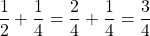Working with larger numbers in adding fractions makes things tougher. Consider eleven out of seventy plus 3 out of 8. One common denominator is 560. This is from 70 x 8 = 560.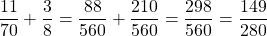Long Division

Long division with division by a single digit number is not too bad. Something like `555 divided by 3` is not too bad with the steps. Larger numbers in long division makes things much tougher. Even though the steps are pretty much the same doing something like `12430 divided by 24` is not so easy compared to `555 divided by 3`.

Algebra Cases

When it comes to algebra and order of operations (BEDMAS/PEDMAS) working with smaller numbers is much easier than working with larger numbers. This is more true if you are not allowed calculator usage.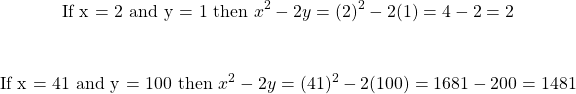Pixabay Image Source

## When Grades Matter For University Applications

With my area near the city of Toronto, grade 12 is an important grade. It is the last year of high school and grades are very important for applying to university and colleges here. Grades in the 80s and 90s are typically competitive enough for many top programs and top universities.

It is kind of scary to think that a certain question with confusing wording can impact the score of a test. This test score can then impact the math course grade and then the overall average for university & college applications.Pixabay Image Source